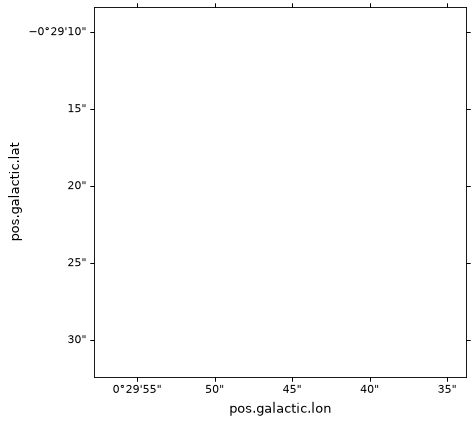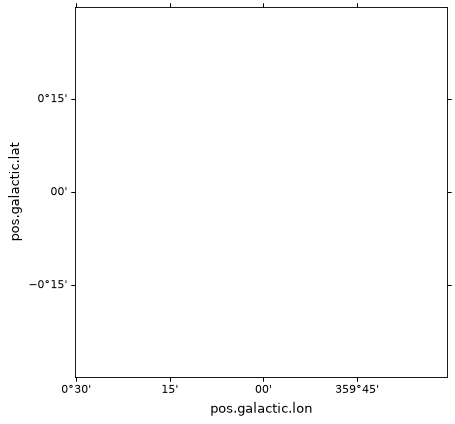# Initializing axes with world coordinates¶

## Basic initialization¶

To make a plot using `WCSAxes`, we first read in the data using astropy.io.fits and parse the WCS information. In this example, we will use an example FITS file from the http://data.astropy.org server (the `get_pkg_data_filename()` function downloads the file and returns a filename):

```from astropy.wcs import WCS
from astropy.io import fits
from astropy.utils.data import get_pkg_data_filename

filename = get_pkg_data_filename('galactic_center/gc_msx_e.fits')

hdu = fits.open(filename)
```

We then create a figure using Matplotlib and create the axes using the `WCS` object created above. The following example shows how to do this with the Matplotlib ‘pyplot’ interface, keeping a reference to the axes object:

```import matplotlib.pyplot as plt
ax = plt.subplot(projection=wcs)
```

(png, svg, pdf)The `ax` object created is an instance of the `WCSAxes` class. Note that if no WCS transformation is specified, the transformation will default to identity, meaning that the world coordinates will match the pixel coordinates.

The field of view shown is, as for standard matplotlib axes, 0 to 1 in both directions, in pixel coordinates. As soon as you show an image (see Plotting images and contours), the limits will be adjusted, but if you want you can also adjust the limits manually. Adjusting the limits is done using the same functions/methods as for a normal Matplotlib plot:

```ax.set_xlim(-0.5, hdu.data.shape - 0.5)
ax.set_ylim(-0.5, hdu.data.shape - 0.5)
```

(png, svg, pdf)Note

If you use the pyplot interface, you can also replace `ax.set_xlim` and `ax.set_ylim` by `plt.xlim` and `plt.ylim`.

## Alternative methods¶

As in Matplotlib, there are in fact several ways you can initialize the `WCSAxes`.

As shown above, the simplest way is to make use of the `WCS` class and pass this to `plt.subplot`. If you normally use the (partially) object-oriented interface of Matplotlib, you can also do:

```fig = plt.figure()
ax = fig.add_subplot(1, 1, 1, projection=wcs)
```

Note that this also works with `add_axes()` and `axes()`, e.g.:

```ax = fig.add_axes([0.1, 0.1, 0.8, 0.8], projection=wcs)
```

or:

```plt.axes([0.1, 0.1, 0.8, 0.8], projection=wcs)
```

Any additional arguments passed to `add_subplot()`, `add_axes()`, `subplot()`, or `axes()`, such as `slices` or `frame_class`, will be passed on to the `WCSAxes` class.

## Directly initializing WCSAxes¶

As an alternative to the above methods of initializing `WCSAxes`, you can also instantiate `WCSAxes` directly and add it to the figure:

```from astropy.wcs import WCS
from astropy.visualization.wcsaxes import WCSAxes
import matplotlib.pyplot as plt

wcs = WCS(...)

fig = plt.figure()
ax = WCSAxes(fig, [0.1, 0.1, 0.8, 0.8], wcs=wcs)
fig.add_axes(ax)  # note that the axes have to be explicitly added to the figure
```